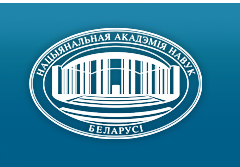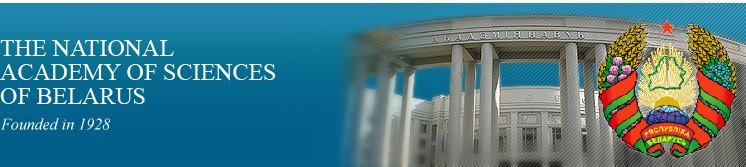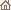Home News About Academy Academy Activities Academy Membership Organizations Publications Conferences Contacts Site Map

# Proc. of the Natl. Academy of Sciences of Belarus, Ser. Phys.-Math. Sci., 2007, No.4/ Publications / Scientific Journals

### Number 4, 2007## CONTENTS

### MATHEMATICS

Rusak V. N., Rovba E. A.
Rational approximation of Stieltjes functions at the real axis. pp. 4--9

Summary: Exact estimates of deviations of Stieltjes analytic in halfplane functions from rational operators of Fourier type have been obtained.

Kashevskij V. V.
Logarithmic integral of periodic functions and its properties. pp. 10--14

Summary: The logarithmic integral and also its connection with Fourier series is considered. The representation of the logarithmic integral as convolution is given. The continuity module of the indicated integral of continuous functions is investigated. The boundedness of the logarithmic integral in Holder spaces is proved.

Malyutin V. B.
Evaluation of multiple functional integrals from the description of particles with a half-integer spin. pp. 15--21

Summary: The multiple functional integrals describing the particles with a half-integer spin are represented by functional integrals over spine variables, and a class of multiple functional integrals is evaluated.

Belsky V. A.
Riccati equations with the same reflective functions. pp. 22--27

Summary: In the paper for the Riccati equations the conditions for considering reflective functions of Mironenko V. I. were established. It is proved that for every Riccati equation one can construct another with the same shift-operator on [-w,w] with the even in t right-hand side.

Nelepko M. S.
Power and exponential expansions of the equations of the КII hierarchy. pp. 28--33

Summary: The equation of the КII hierarchy is considered, which is obtained, when n = 2. Its Newton polygon is the same as the 6P2 Painleve equation has. The Newton polygon method is applied to obtain all power expansions of the solutions near the points z = 0 and z = ¥. The exponential additions to the expansions near z = ¥ are found.

Kavalchuk A. N., Yablonski A. L.
Asymptotic behavior of the finite sums with the approximations of the Brownian motion. pp. 34--39

Summary: The necessary and sufficient conditions for convergence of the sums with the aрproximations of the Brownian motion are obtained. The estimates of the convergence degree are found.

Safonov V. G.
Totally saturated formations with metanilponent l¥-defect 3. pp. 40--46

Summary: The article presents the description of totally saturated formations of metanilpotent l¥-defect 3.

Gribovskaya M. A., Monakhov V. S.
Subnormal subgroups and indices of maximal subgroups of finite groups. pp. 47--50

Summary: Let K be subnormal subgroup of a group G of odd order. Suppose that the indices of all maximal subgroups, which are not divided into subgroup K, the fifth degree of prime numbers. It is proved that the second derived subgroup K is nilpotent.

Myaleshka I. N.
Approximate solution of the base limit problem for the biharmonic function in the circle. pp. 51--55

Summary: An approximate presentation of the solution of the base limit problem for the biharmonic function in the circle by the logarithms is considered.

Bondarenko A. A.
Birational composition of quadratic forms. pp. 56--61

Summary: Let f(X) and g(Y) be nonsingular quadratic forms over the field K having dimensions m and n, char K ¹ 2. In some cases, we get a positive answer to the question: when is the product f(X)g(Y) over K birationally equivalent to the quadratic form h(Z) over K having dimensions m + n? The examples of the absence of h(Z) are given.

Vasilyev D. V., Kukso O. S.
Distribution of the values for the distance between the plane points and the algebraic points. pp. 62--66

Summary: The article is devoted to the proof of the analogue of the divergence case of Khintchine theorem for simultaneous approximations for R2.

Leonov N. N.
e-Entropy of functional classes in ultrametric spaces. pp. 67--73

Summary: The order of growing e-entropy of a class of functions, which map one compact homogeneous ultrametric space onto another and satisfy the continuity module restriction, is found.

Naidenko V. G.
Topological properties of half-spaces of directional convexity. pp. 74--78

Summary: A problem of simple connectivity of half-spaces of directional convexity is investigated. We have proved that there exists a connected half-space of ortho-convexity in the three-dimensional space, which is not simply connected.

### PHYSICS

Sotsky A. B., Sotskaya L. I.
Diffraction of a focused light beam by 2D surface defects. pp. 79--83

Summary: The method based on the circular Fourier analysis of TE- and TM-waves for computing the diffracted fields, which are realized when 2D surface defects are illuminated with a focused light beam, is proposed. A correlation of the far field intensity angular diagrams with the shape of a microscopic step on a Si surface is investigated.

Ilyina E. A., Kushnir V. N., Prischepa S. L.
Boundary effects in superconductor/normal metal three-layered mesoscopic structures. pp. 84--88

Summary: The problem on identification of parameters of three-layered Cu/Nb/Cu and Nb/Cu/Nb structures is concerned. It is shown that in the last case the presence of free outer edges of superconductor leads to the distortion of the material parameters that describe the properties of this structure.

Solovtsov I. L.†, Solovtsova O. P.
Vacuum condensates in the variational perturbation theory in quantum chromodynamics. pp. 89--94

Summary: A nonperturbative method based on the variational perturbation theory has been developed. Borel type sum rules allowing us to determine the rest parts of condensates have been constructed. It has been shown that within the method suggested the optimal values of these lower dimensional condensates are compatible with zero.

Karolik A. S.
Electrical resistivity of grain boundaries in compact submicro- and nanostructures. pp. 95--100

Summary: On the basis of the grain boundaries model previously elaborated for polycrystals the change of electrical resistivity per unit grain boundaries density rGnano / NG for compact nanocrystalline monovalent and transitional metals is calculated using the partial-wave method. It is supposed that the grain boundaries in nanostructures have preferentially high-angle misorientations and are characterized by increased dilatation of a lattice as a result of nonequilibrium and high elastic distortions of a lattice. The calculated values rGnano / NG are found to be by a factor of one and a half - two higher than the corresponding values for polycrystals. In the lack of experimental data for nanocrystalline metals, the calculation results are compared with the available data for compact submicrocrystalline metals obtained by severe plastic deformation with a mean size of grains from 100 up to 250 nanometers.

Nemtsov V. B., Shirko A. V., Kamluk A. N.
Thermodynamic description of a B-S-transition at overstretching of DNA molecule. pp. 101--105

Summary: On the basis of the thermodynamic description of nonlinear overstretching of DNA molecule it is shown that the force-deformation equation is similar to the van der Waals equation. The B-DNA to S-DNA transition is considered as the stability breaking. The coexistence and spinodal curves are obtained. The position of the accompanied plateau is determined by means of the Maxwell rule analogously to the case of the van der Waals equation. The «critical» point for the overstretching of DNA molecule is predicted.

### INFORMATICS

Tiunchik A. A.
Systolic realization of the multiplication algorithm of a point on the elliptic curve. pp. 106--112

Summary: A systolic array capable to carry out the addition, multiplication and squaring of elements of the field GF( 2k ) by the Montgomery algorithm is designed using the systematic approach. Realization of these operations can be carried out in any (unknown beforehand) sequence determined by a specific realization of an algorithm of multiplication of a point on an elliptic curve. The device of the processor does not depend either on a number of the operations or on their sequence.

Benediktovich V. I.
Noncrossing spanning tree of geometric complement of the plane Hamiltonian cycle. pp. 113--117

Summary: In this article the criterion for existence of a noncrossing spanning tree in the geometric complement of the plane has been obtained.

Gvozd E. N., Minchenko L. I.
Different types of constraint qualification in parametrical mathematical programming. pp. 118--122

Summary: A constraint qualification of constant rank and a metrical regularity are studied. It is proved that the constant rank constraint qualification implies the metric regularity condition.

### SCIENTISTS OF BELARUS

Aleksei Petrovich Shkadarevich (To the 60th Anniversary). pp. 123--124
Click
here to read this article in Russian (Win 1251)/ Publications / Scientific Journals / Back to Top of Page

Designed and maintained by Dr. Nikolai N. Kostyukovich. Last updated: December 13, 2007
Created with assistance of Dr. Ignatii I. Korsak
Copyright © 2007 The National Academy of Sciences of Belarus
Copyright © 2007 The Belaruskaya Navuka Publishing House